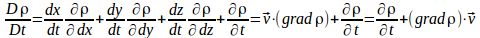## Alternative Forms of The Continuity Equation

The continuity equation is usually written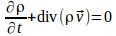(1) where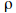is the density of the fluid at a point.

< >It may also be written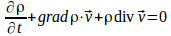(2) or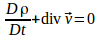(3) where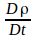is the Stokes derivative.

< >(2) follows from (1) on using the identity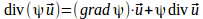< >To derive (3) write